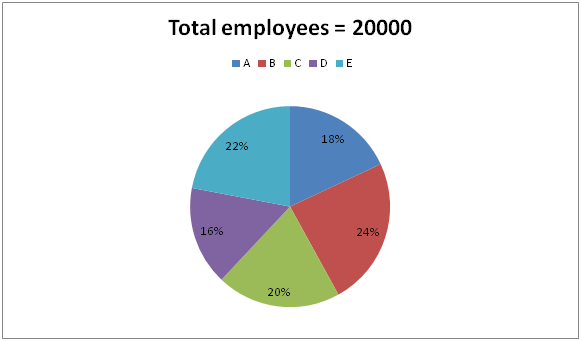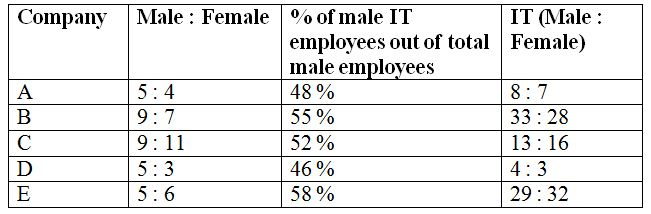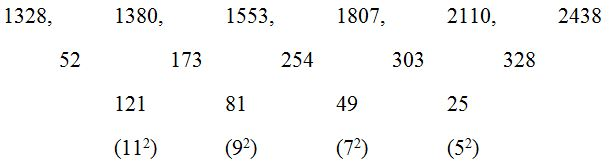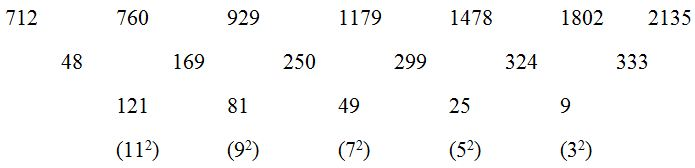# SBI Clerk/ Repco/ IDBI and South Indian Bank Mains Quantitative Aptitude Questions 2019 (Day-01)

Dear Aspirants, Our IBPS Guide team is providing a new series of Quantitative Aptitude Questions for SBI Clerk /Repco/IDBI and South Indian Bank Mains 2019 so the aspirants can practice it on a daily basis. These questions are framed by our skilled experts after understanding your needs thoroughly. Aspirants can practice these new series questions daily to familiarize with the exact exam pattern and make your preparation effective.

Check here for IBPS RRB Clerk Prelims Mock Test 2019

Check here for EPFO Assistant Mock Test 2019

Check here for LIC ADO Mock Test 2019

[WpProQuiz 6584]

Directions (Q. 1 – 5): The questions below are based on the given Series-I. The series-I satisfy a certain pattern, follow the same pattern in Series-II and answer the questions given below.

1) I) 13, 7, 8, 18, 76, 616

II) 25 ……. 16016. If 16016 is nth term, then what value should come in place of (n – 2)th term?

a) 132

b) 156

c) 124

d) 148

e) 160

2) I) 1328, 1380, 1553, 1807, 2110, 2438

II) 712, 760 ……. 2135. If 2135 is nth term, find the value of n?

a) 7

b) 8

c) 6

d) 5

e) 4

3) I) 12, 16, 41, 139, 581, 2941

II) 30 ……. 5101. If 5101 is nth term, then what value should come in place of (n+1)th term?

a) 20565

b) 22723

c) 26148

d) 30655

e) 18352

4) I) 972, 324, 108, 36, 12, 4

II) 9477 …… 13. If 13 is nth term, then what value should come in place of (n – 3)rd term?

a) 417

b) 443

c) 351

d) 375

e) 526

5) I) 254, 318, 1047, 1147, 2478, 2622

II) 118 …… 4683. If 4683 is nth term, then find the value of n?

a) 8

b) 7

c) 6

d) 5

e) 4

Directions (Q. 6 – 10): Study the following information carefully and answer the given questions?

The following pie chart shows the total number of employees (HR + IT department) working in 5 different companies.The table shows the ratio of male to that of female employees and the percentage of male IT employees and the ratio of male to that of female IT employees working in those companies.6) Find the ratio between the total number of female IT employees in company A and C together to that of total number of HR male employees in the same companies together?

a) 249 : 238

b) 152 : 73

c) 45 : 11

d) 377 : 195

e) None of these

7) Find the difference between the total number of HR employees working in company B to that of company D?

a) 570

b) 625

c) 380

d) 465

e) None of these

8) Total number of male employees in company A and E together is approximately what percentage of total number of male employees in company C and D together?

a) 130 %

b) 105 %

c) 145 %

d) 90 %

e) 75 %

9) Find the total number of HR employees in company C, D and E together?

a) 4878

b) 5044

c) 5462

d) 5236

e) None of these

10) In which of the following companies, the second highest number of male employees working in IT department?

a) Company A

b) Company B

c) Company C

d) Company D

e) Company E

Direction (1-5 ):

Series I pattern:

13 * 0.5 + 0.5 = 7

7 * 1 + 1 = 8

8 * 2 + 2 = 18

18 * 4 + 4 = 76

76 * 8 + 8 = 616

Series II pattern:

25 * 0.5 + 0.5 = 13

13 * 1 + 1 = 14

14 * 2 + 2 = 30

30 * 4 + 4 = 124 (n – 2)th term

124 * 8 + 8 = 1000 (n – 1)th term

1000 * 16 + 16 = 16016 (n)th term

Series I pattern:The difference of difference is, 112, 92, 72, 52, ….

Series II pattern:

712 is first term.2195 is 7th term. So, n = 7

Series I pattern:

12 * 1 + 22 = 16

16 * 2 + 32 = 41

41 * 3 + 42 = 139

139 * 4 + 52 = 581

581 * 5 + 62 = 2941

Series II pattern:

30 * 1 + 22 = 34

34 * 2 + 32 = 77

77 * 3 + 42 = 247

247 * 4 + 52 = 1013

1013 * 5 + 62 = 5101 (nth) term

5101 * 6 + 72 = 30655 (n + 1)th term

Series I pattern:

972 ÷ 3 = 324

324 ÷ 3 = 108

108 ÷ 3 = 36

36 ÷ 3 = 12

12 ÷ 3 = 4

Series II pattern:

9477 ÷ 3 = 3159

3159 ÷ 3 = 1053

1053 ÷ 3 = 351 (n – 3)rd term

351 ÷ 3 = 117 (n – 2)th term

117 ÷ 3 = 39 (n – 1)th term

39 ÷ 3 = 13 (nth term)

Series I pattern:

254 + 82 = 318

318 + 93 = 1047

1047 + 102 = 1147

1147 + 113 = 2478

2478 + 122 = 2622

Series II pattern:

118 is first term

118 + 82 = 182

182 + 93 = 911

911 + 102 = 1011

1011 + 113 = 2342

2342 + 122 = 2486

2486 + 133 = 4683

4683 is 7th term. So, n = 7

Direction (6-10) :

The total number of female IT employees in company A and C together

= > 20000*(18/100)*(5/9)*(48/100)*(7/8) + 20000*(20/100)*(9/20)*(52/100)*(16/13)

= > 840 + 1152 = 1992

The total number of HR male employees in company A and C together

= > 20000*(18/100)*(5/9)*(52/100) + 20000*(20/100)*(9/20)*(48/100)

= > 1040 + 864 = 1904

Required ratio = 1992 : 1904 = 249 : 238

The total number of HR employees working in company B

= > 4800 – [4800*(9/16)*(55/100)*(61/33)]

= > 4800 – 2745 = 2055

The total number of HR employees working in company D

= > 3200 – [3200*(5/8)*(46/100)*(7/4)]

= > 3200 – 1610 = 1590

Required difference = 2055 – 1590 = 465

Total number of male employees in company A and E together

= > 3600*(5/9) + 4400*(5/11)

= > 2000 + 2000 = 4000

Total number of male employees in company C and D together

= > 4000*(9/20) + 3200*(5/8)

= > 1800 + 2000 = 3800

Required % = (4000/3800)*100 = 105.26 % = 105 %

The total number of HR employees in company C, D and E together

= > {4000 – [4000*(9/20)*(52/100)*(29/13)]} + {3200 – [3200*(5/8)*(46/100)*(7/4)]} + {4400 – [4400*(5/11)*(58/100)*(61/29)]}

= > {4000 – 2088} + {3200 – 1610} + {4400 – 2440}

= > 1912 + 1590 + 1960 = 5462

In company A

= > 3600*(5/9)*(48/100) = 960

In company B

= > 4800*(9/16)*(55/100) = 1485

In company C

= > 4000*(9/20)*(52/100) = 936

In company D

= > 3200*(5/8)*(46/100) = 920

In company E

= > 4400*(5/11)*(58/100) = 1160

In company E, the second highest number of male employees working in IT department.